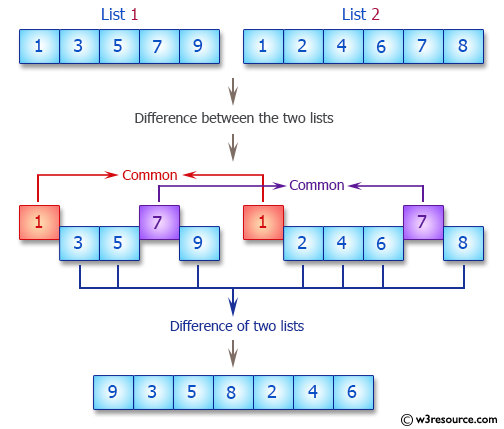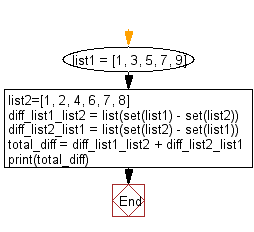﻿ Python: Difference between the two lists - w3resource# Python: Difference between the two lists

## Python List: Exercise - 19 with Solution

Write a Python program to get the difference between the two lists.Sample Solution:-

Python Code:

``````list1 = [1, 3, 5, 7, 9]
list2=[1, 2, 4, 6, 7, 8]
diff_list1_list2 = list(set(list1) - set(list2))
diff_list2_list1 = list(set(list2) - set(list1))
total_diff = diff_list1_list2 + diff_list2_list1
print(total_diff)
```
```

Sample Output:

```[9, 3, 5, 8, 2, 4, 6]
```

Flowchart:## Visualize Python code execution:

The following tool visualize what the computer is doing step-by-step as it executes the said program:

Python Code Editor:

Have another way to solve this solution? Contribute your code (and comments) through Disqus.

What is the difficulty level of this exercise?

Test your Python skills with w3resource's quiz

﻿

## Python: Tips of the Day

Floor Division:

When we speak of division we normally mean (/) float division operator, this will give a precise result in float format with decimals.

For a rounded integer result there is (//) floor division operator in Python. Floor division will only give integer results that are round numbers.

```print(1000 // 300)
print(1000 / 300)```

Output:

```3
3.3333333333333335```